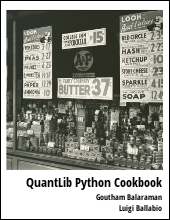Provides an introduction to valuation of treasury futures contract in QuantLib Python.

Visit here for other QuantLib Python examples. If you found these posts useful, please take a minute by providing some feedback.

In this post, we will learn how to value treasury futures contract using QuantLib. The treasury futures contract gives the buyer the right to buy the underlying by the time the contract expires. The underlying is usually delivered from a basket of securities. So in order to properly value the futures contract, we would need to find the deliverable. Here we start by doing a naive calculation by constructing a fictional security. We will see what is wrong about this approach. As a next step we will perform the cheapest to deliver calculation and subsequently use that deliverable to value the same contract.

In :
import QuantLib as ql
import math

In :
calc_date = ql.Date(30,11,2015)
ql.Settings.instance().evaluationDate = calc_date
day_count = ql.ActualActual()
calendar = ql.UnitedStates()
bussiness_convention = ql.Following
end_of_month = False
settlement_days = 0
face_amount = 100
coupon_frequency = ql.Period(ql.Semiannual)


## Build Yield Curve

As a first step, we build the treasury curve out of the treasury securities such as T-Bills, T-Notes and Treasury bonds.

In :
Expand Code
In :
yield_curve = ql.PiecewiseCubicZero(calc_date, bond_helpers, day_count)
yield_curve_handle = ql.YieldTermStructureHandle(yield_curve)
for d in maturity_dates:
print "|%20s  %9.6f|"%(d, yield_curve.discount(d))

| December 24th, 2015   0.999935|
| February 25th, 2016   0.999576|
|      May 26th, 2016   0.998119|
| November 10th, 2016   0.995472|
| November 30th, 2017   0.981524|
| November 15th, 2018   0.964278|
| November 30th, 2020   0.920306|
| November 30th, 2022   0.868533|
| November 15th, 2025   0.799447|
| November 15th, 2045   0.384829|


## Treasury Futures

Here we want to understand how to model treasury futures contract. Let us look at the TYZ5, the treasury futures on the 10 year note for delivery in December 2015. The notional deliverable is a 10-year 6% coupon note. In reality, the seller of the futures contract can deliver from a basket of securities.

For now, lets assume that the deliverable is actually a 6% coupon 10-year note issued as of the calculation date. Let us construct a 10 year treasury note and value this security. The futures price for the TYZ5 is $127.0625. In : Expand Code In : bond_issue_date = calc_date delivery_date = ql.Date(1,12,2015) bond_maturity_date = bond_issue_date + ql.Period(10, ql.Years) day_count = ql.ActualActual() coupon_frequency = ql.Period(6, ql.Months) coupon_rate = 6/100. deliverable = create_tsy_security(bond_issue_date, bond_maturity_date, coupon_rate, coupon_frequency, day_count, calendar ) bond_engine = ql.DiscountingBondEngine(yield_curve_handle) deliverable.setPricingEngine(bond_engine)  Lets calculate the Z-Spread for this deliverable. The Z-Spread is the static spread added to the yield curve to match the price of the security. This spread is a measure of the risk associated with the security. For a treasury security, you would expect this to be zero. In : futures_price = 127.0625 clean_price = futures_price*yield_curve.discount(delivery_date) zspread = ql.BondFunctions.zSpread(deliverable, clean_price, yield_curve, day_count, ql.Compounded, ql.Semiannual, calc_date)*10000 print "Z-Spread =%3.0fbp" % (zspread)  Z-Spread = 71bp  Here we get a spread of 71 basis points. This is unusually high for a treasury futures contract. ### Cheapest To Deliver Above we used a fictional 6% coupon bond as the deliverable. In reality, the deliverable is picked from a basket of securities based on what is the cheapest to deliver. Cheapest to deliver is not the cheapest in price. The seller of the futures contract, has to buy the delivery security from the market and sell it at an adjusted futures price. The adjusted futures price is given as: Adjusted Futures Price = Futures Price x Conversion Factor The gain or loss to the seller is given by the basis, Basis = Cash Price - Adjusted Futures Price So the cheapest to deliver is expected to be the security with the lowest basis. The conversion factor for a security is the price of the security with a 6% yield. Let us look at a basket of securities that is underlying this futures contract to understand this aspect. In : Expand Code Minimum Basis = 0.450601 Conversion Factor = 0.791830 Coupon = 2.125000 Maturity = June 30th, 2022 Price = 101.062500  The basis is the loss for a notional of \$100 that the seller accrues to close this contract. For a single futures contract (which has a \$100000 notional), there is a loss of \$450.60.

NOTE: You will need my pull request to execute the FixedRateBondForward class since it is not exposed in SWIG at the moment.

In :
futures_maturity_date = ql.Date(21,12,2015)
futures = ql.FixedRateBondForward(calc_date, futures_maturity_date,
ql.Position.Long, 0.0, settlement_days,
day_count, calendar, bussiness_convention,
ctd_bond, yield_curve_handle, yield_curve_handle)


The valuation of the futures contract and the underlying is shown below:

In :
Expand Code
Model Futures Price            = 127.610365
Market Futures Price           = 127.062500
Implied Yield                  = -7.473%
Forward YTM                    = 1.952%


 Understanding Treasury Futures, CME Group PDF

## Conclusion

In this notebook, we looked into understanding and valuing treasury futures contracts. We used the QuantLib FixedRateBondForward class in order to model the futures contract. But, we also made a cheapest to deliver calculation to figure out the deliverable.